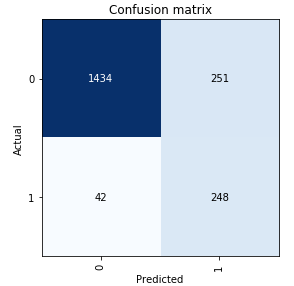# Weird top loss with weight

Hi,

My problem is similar to Inconsistency in interp.plot_top_losses, but i am sure I have enough wrong classification to avoid the problem that he was having.

I have modified the notebook from lesson 4 to classify paper abstracts. Here is my confusion matrix:But here is my top loss:

You can see that the top one has high loss, but classification is 100% probability of correct result. I am trying to understand why. The only thing that I might have done differently is that I have to set loss weight to account for imbalanced data:

# when creating learner

weight = tensor(1., 4).cuda();
learn.loss_func = CrossEntropyFlat(weight=weight)

# when creating interpreter

learn.loss_func = CrossEntropyFlat(weight=tensor(1., 4))
interp = TextClassificationInterpretation.from_learner(learn)

Any help will be highly appreciated.